## Fibonacci Benchmark

The purpose of this Benchmark is to test the Fibonacci Function using recursive coding and parallel programming. For more information about the benchmark go to: CPU Performance - Part 1 and CPU Performance - Part 2
The Following Programs are discussed:
1. VB Fibonacci50. This is a Fibonacci Program written in Visual Basic 5.0
2. Fibonacci. This is a Fibonacci Program written in Visual Basic 2010
3. FibonacciPP. This is a Fibonacci Program written in Visual Basic 2010 and supports parallel programming.
To get a copy of the Visual Basic 5.0 .exe file select VB exe.zip.
To get a copy of the Visual Basic 2010 .exe files select VB2010 exe.zip.

## Introduction Fibonacci Numbers

 The Fibonacci numbers are generated using the following recursive function: Fn1 = Fn-1 + Fn-2 (1)
1. In the case of one processor you can directly implement the recursive function: Fn = Fn-1 + Fn-2
2. In the case with two processors you follow a slightly different path:
• In the first processor you calculate the Fibonacci function: Fn-1
• In the second processor you calculate the Fibonacci function: Fn-2
• When the two are finished you perform the calculation Fn = Fn-1 + Fn-2
3. When you want to perform a Benchmark testing parallel programming then the basic rule should be that the mathematics used with or without parallel programming should be identical.
The problem is 1 and 2 are not identical.
4. In the case of one processor you should perform the following.
• First you calculate the Fibonacci function: Fn-1
• Secondly you calculate the Fibonacci function: Fn-2
• And finally you perform the calculation Fn = Fn-1 + Fn-2
When you compare 2 and 4 the two are mathematically identically. This strategy of load sharing is followed in the program FibonacciPP

 In the case of three processors the Fibonacci Function is: Fn = Fn-2 + 2 * Fn-3 + Fn-4 (2) In the case of four processors the Fibonacci Function is: Fn = Fn-3 + 3*Fn-4 + 3*Fn-5 + Fn-6 (3) In the case of five processors the Fibonacci Function is: Fn = Fn-4 + 4*Fn-5 + 6*Fn-6 + 4*Fn-7 + Fn-8 (4)
Equation 4 the function implemented in the programme: FibonacciPP
That means first the numbers Fn-4, Fn-5, Fn-6, Fn-7 and Fn-8 are calculated (in parallel) and finally the number Fn is calculated using equation 4.
Load sharing in the different processors is as follows

 # of P Fn-4 Fn-5 Fn-6 Fn-7 Fn-8 1 1 1 1 1 1 2 1 2 2 1 1 3 1 2 3 3 2 4 1 2 3 4 4 5 1 2 3 4 5

## 1 Program VB Fibonacci50

The program "VB Fibonacci50" is written in Visual Basic 5.0, calculates Fibonacci numbers using equation (1) and does not support parallel programming.

## 1.1 Operation "VB Fibonacci50" & results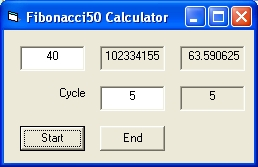### Pentium 4 2.8 Ghz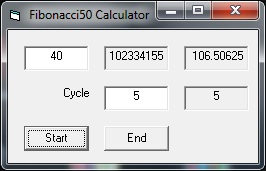### i5 2C/4T

The results show that the i5 with one processor (25% load) is slow compared to the P4 2.8

## 2 Program Fibonacci

The program Fibonacci is written in Visual Basic 2010, calculates Fibonacci numbers using equation 1 and does not support parallel programming.
The program was based on the Fibonacci Example in the Microsoft MSDN Document: BackGroundWorker. The microsoft program consists of one Visual Basic program while standard in the Visual Basic 2010 environment there are 2. See Visual Basic 2010 evaluation for details about documentation issues.
In the Fibonacci microsoft document they moved all the fibonacci.designer.vb program code into the fibonacci.vb program. That seems smart if you are a professional but it makes it very difficult for the average user. Specific to make changes.
A cycle counter is introduced to improve accuracy
The code with controls the bar is also changed. In the microsoft program the bar is almost updated continuous. That is a very time consuming operation specific if you want to test the performance in different processors. (See FibonacciPP). The bar is only updated at the end of each cycle.

Fibonacci Application listings: FibonacciForm.vb and FibonacciForm.Designer.vb

## 2.1 Operation Fibonacci & results### Pentium 4 2.8 Ghz### i5 2C/4T

The results show that the i5 with one processor (25% load) is slow compared to the P4 2.8

## 3 Program FibonacciPP

The program FibonacciPP is written in Visual Basic 2010, calculates Fibonacci numbers, supports parallel programming and uses equations (2), (3) and (4).
See Visual Basic 2010 Parallel Programming techniques The Master does not use the Backgroundworker. Each of the slaves uses a Backgroundworker

## 3.1 Operation FibonacciPP

When you start the program the form shows the following parameters and buttons:
1. In the top left corner the number n tested. The minimum number is 9.
2. In the midle the Fibonacci value calculated.
3. In the top right corner the time in seconds.
4. The next line shows the progres bar.
5. Next to the text "# Pr" the number of processors tested. The value can between 1 and 5.
6. Next to the text "Cycle" the number of cycles tested. The minimal number is 1.
7. The next box shows the actual number of cycles tested in progress.
8. The pushbotton "Start Async"is used to start the calculation.
9. The pushbotton "Cancel Async"is used to terminate the calculation in porgress.

FibonacciPP Application listings: FibonacciPPForm.vb and FibonacciPPForm.Designer.vb

## i5 CPU Performance 2C/4T - FibonacciPP Simulation

The following displays show the results for n = 40.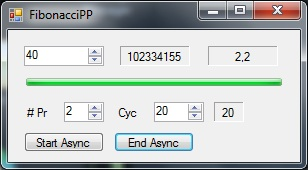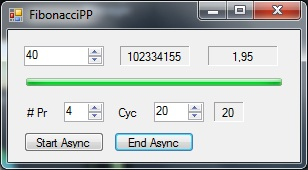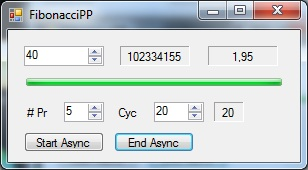The following displays show the results for n= 45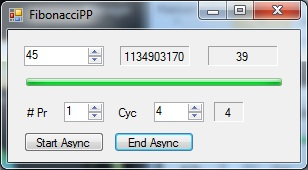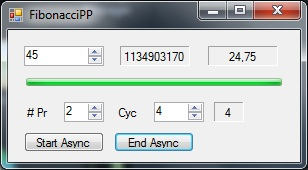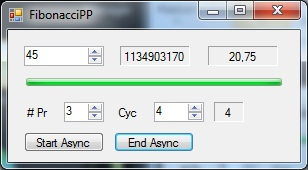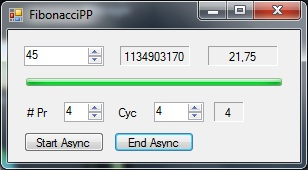For n = 40 for processors 1 to 5 the results are: 3.5 2.1 1.9 1.95 and 1.95
For n = 45 for processors 1 to 5 the results are: 39 24.75 20.75 21.75 and 22.25
The load numbers are roughly 25%,50%,75%, 100% and 100%
What the results show is that the best result is for 3 processors, however that this is small.

## P4 2.8 Performance 1C/1T - FibonacciPP Simulation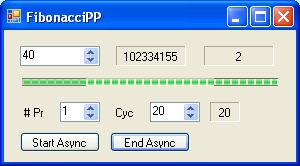The results are for n=40 : 2 and for n=45: 22.25

## Reflection

CPU 1 is a "two core" / "4 thread" i5 CPU, which supports parallel programming.
CPU 2 is a "single core" / "1 thread" CPU and which does not support parallel programming

If you compare the results of CPU 1 which CPU 2 than the results of CPU 2 are very good. This is rather remarkable.

E-mail:nicvroom@telenet.be.

Updated: 10 August 2012
Updated: 4 November 2014

Back to CPU Performance - Benchmark part 2
Back to my home page Contents of This Document